12. Material-Ideal Time

L. G. Kreidik (translation from Russian T. S. Kortneva and G. P. Shpenkov)

12.1. Introduction

Fundamental ideal property of motion-rest of any level is length of motion-rest. A measure of the length, or a measure of duration, is the quantitative side of objective physical time. This aspect in human consciousness is reflected as subjective intuitive quantitative time.

Qualitative and quantitative aspects of time are related inseparably because objective time is the quantitative-qualitative field of the Universe. Objective time is represented by an infinite series of ideal levels of the Universe and it is nonuniform on all levels, though it is generally thought to be uniform.

Classical physics operates with standard imaginary time that does not exist in the objective world and only exists in the subjective space of our thought. Our thought, however, belongs to the Universe and is realized in its, therefore, in this sense the standard time is also objective.

It is possible to create a timer with a certain accuracy which simulates subjective uniform time and to declare it to be world Time. The course of the timer's time can change but the subjective uniform time entering in equations and describing physical processes must not be changed. That does not exist in objective reality cannot become longer or shorter.

We will consider dialectics of physical time, comparing it with uniform subjective ideal time. While speaking about time, it is necessary to distinguish the general notion of time and the notion of time of a particular process. We will be interested primarily in concrete time and the main components of its structure.

Since any object in nature is a rest-motion system, its time should be potential-kinetic. This means that time is contradictory and has components of rest and motion, i.e. it contains time of rest and time of motion. These times are qualitatively different.

The time of rest is the potential time and its should be described by a quantitative numerical field because this field is used for description of potential displacement. The time of motion is kinetic and should be expressed by a qualitative numerical field as kinetic displacement.

12.2. A measure of physical time for harmonic displacement

So, it is natural that the length of rest-motion is taken as a measure of the physical time. A qualitative measure of physical time have to describe also the form of rest-motion. Both measures have to be multiplicatively joined in unit dialectic measure of time.

On the level of potential-kinetic harmonic displacement, the physical time should follow motion-rest with some amplitude.

In a harmonic oscillation the character of change of motion-rest is described by the exponent of standard time and objective time should be an ideal time field with the same change. Therefore, the expression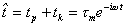, (2.279)

can be a measure of objective potential-kinetic time.

In this expression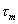is the time amplitude whose measure is determined by the relation relating the displacement y with the velocity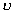;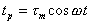, (2.280)

it replicates the structure of a potential displacement and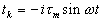, (2.281)

which has the analogous structure as a kinetic displacement.

Potential-kinetic time of a harmonic displacement is a local oscillating wave of time defining the displacement: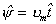, (2.282)

where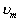is the velocity amplitude.

In addition, the potential and kinetic components of the displacement and time are related by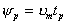,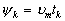. (2.283)

Formulae (2.282) and (2.283) show that physical time is a scalar-vector quantity.

If we take the amplitude value of displacement a and velocity, we obtain the amplitude of time: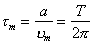, (2.284)

which, as a quantitative measure, is simultaneously a potential amplitude of time.

The ratio of the instantaneuos displacement to the instantaneous velocity gives the kinetic amplitude of time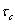: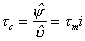(2.285)

A quarter of the standard time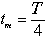(2.286)

can be taken as a measure of the amplitude of physical time. This measure determines a maximum deviation of the material point from the equilibrium position. With this measure of the amplitude, the average potential time is equal to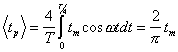(2.287)

and related with the amplitude of time tm just in the same way as the average potential displacement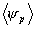is related with the displacement amplitude a: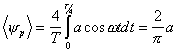(2.288)

to the time amplitude in expression (2.284).

If the time is defined by amplitude (2.286), the ratio of the displacement V to time t is equal to the average kinetic velocity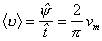(2.289)

and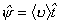. (2.290)

In this case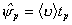,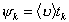. (2.291)

Objective time is characterized by its field of velocity of time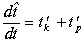, (2.292)

where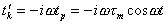(2.293)

is the kinetic velocity of time and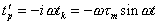(2.294)

is the potential velocity of time.

Comparison of the kinetic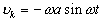and potential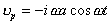velocities with the corresponding velocities of time shows that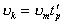,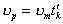(2.295)

and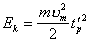,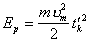. (2.296)

Thus, kinetic energy of harmonic oscillation is proportional to square of the potential velocity of time. In this sense, the kinetic energy of displacement is the potential energy of time. Correspondingly, the potential energy of harmonic oscillation is proportional to square of kinetic velocity of time. It means that the potential energy of displacement is the kinetic energy of time.

12.3. A measure of time in circular and rectilinear motion

Now, we will consider circular time. The circular time is set up by a vector sum of times along Cartesian coordinates x and y with unit vectors k and l: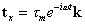,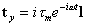, (2.297)

which form the circular time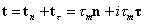. (2.298)

The normal component of the time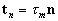(2.299)

is the time of centripetal rest, while the tangential component of time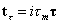(2.300)

is the time of tangential motion.

Both times are supplemented with the axial time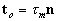. (2.301)

This temporal triad determines the elementary field of time not only in circular but also in rectilinear motion.

Obviously, in circular motion, the relation between the length of the ideal temporal circle and its radius is similar to the analogous relation for the space circle, whose radius is equal to the amplitude of harmonic oscillations forming the circular motion. Since the length of the temporal circle is equal to the ideal period T, its radius is simultaneously the time amplitude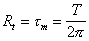. (2.302)

12.4. A general formula of potential-kinetic time

All above time amplitudes are inversely proportional to the specific velocity CO and the general formula of potential-kinetic time becomes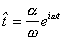, (2.303)

where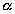is a factor depending on the choice of the measure.

This formula can be a basis for introduction of more general measures of physical time. In particular, if the amplitude of oscillation of a material point changes with the constant specific velocity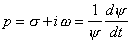(2.304)

the potential-kinetic displacement has the form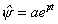, (2.305)

where a is the initial amplitude. In that case, the quantity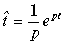, (2.306)

can be used as a measure of time. The displacement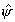will be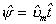, (2.307)

where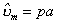is a complex amplitude of velocity.

With this definition of the measure of time, the velocity of time is equal to the generalized exponent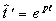. (2.308)

Theoretical Dialectical Journal: Physics-Mathematics-Logic-Philosophy, N.2, site http://www.tedial.narod.ru/